$\newcommand{\NN}{\mathbb{N}} \newcommand{\CC}{\mathbb{C}} \newcommand{\GG}{\mathbb{G}} \newcommand{\LL}{\mathbb{L}} \newcommand{\PP}{\mathbb{P}} \newcommand{\QQ}{\mathbb{Q}} \newcommand{\RR}{\mathbb{R}} \newcommand{\VV}{\mathbb{V}} \newcommand{\ZZ}{\mathbb{Z}} \newcommand{\FF}{\mathbb{F}} \newcommand{\KK}{\mathbb{K}} \newcommand{\UU}{\mathbb{U}} \newcommand{\EE}{\mathbb{E}} \newcommand{\Aa}{\mathcal{A}} \newcommand{\Bb}{\mathcal{B}} \newcommand{\Cc}{\mathcal{C}} \newcommand{\Dd}{\mathcal{D}} \newcommand{\Ee}{\mathcal{E}} \newcommand{\Ff}{\mathcal{F}} \newcommand{\Gg}{\mathcal{G}} \newcommand{\Hh}{\mathcal{H}} \newcommand{\Ii}{\mathcal{I}} \newcommand{\Jj}{\mathcal{J}} \newcommand{\Kk}{\mathcal{K}} \newcommand{\Ll}{\mathcal{L}} \newcommand{\Mm}{\mathcal{M}} \newcommand{\Nn}{\mathcal{N}} \newcommand{\Oo}{\mathcal{O}} \newcommand{\Pp}{\mathcal{P}} \newcommand{\Qq}{\mathcal{Q}} \newcommand{\Rr}{\mathcal{R}} \newcommand{\Ss}{\mathcal{S}} \newcommand{\Tt}{\mathcal{T}} \newcommand{\Uu}{\mathcal{U}} \newcommand{\Vv}{\mathcal{V}} \newcommand{\Ww}{\mathcal{W}} \newcommand{\Xx}{\mathcal{X}} \newcommand{\Yy}{\mathcal{Y}} \newcommand{\Zz}{\mathcal{Z}} \newcommand{\al}{\alpha} \newcommand{\la}{\lambda} \newcommand{\ga}{\gamma} \newcommand{\Ga}{\Gamma} \newcommand{\La}{\Lambda} \newcommand{\Si}{\Sigma} \newcommand{\si}{\sigma} \newcommand{\be}{\beta} \newcommand{\de}{\delta} \newcommand{\De}{\Delta} \renewcommand{\phi}{\varphi} \renewcommand{\th}{\theta} \newcommand{\om}{\omega} \newcommand{\Om}{\Omega} \renewcommand{\epsilon}{\varepsilon} \newcommand{\Calpha}{\mathrm{C}^\al} \newcommand{\Cbeta}{\mathrm{C}^\be} \newcommand{\Cal}{\text{C}^\al} \newcommand{\Cdeux}{\text{C}^{2}} \newcommand{\Cun}{\text{C}^{1}} \newcommand{\Calt}{\text{C}^{#1}} \newcommand{\lun}{\ell^1} \newcommand{\ldeux}{\ell^2} \newcommand{\linf}{\ell^\infty} \newcommand{\ldeuxj}{{\ldeux_j}} \newcommand{\Lun}{\text{\upshape L}^1} \newcommand{\Ldeux}{\text{\upshape L}^2} \newcommand{\Lp}{\text{\upshape L}^p} \newcommand{\Lq}{\text{\upshape L}^q} \newcommand{\Linf}{\text{\upshape L}^\infty} \newcommand{\lzero}{\ell^0} \newcommand{\lp}{\ell^p} \renewcommand{\d}{\ins{d}} \newcommand{\Grad}{\text{Grad}} \newcommand{\grad}{\text{grad}} \renewcommand{\div}{\text{div}} \newcommand{\diag}{\text{diag}} \newcommand{\pd}{ \frac{ \partial #1}{\partial #2} } \newcommand{\pdd}{ \frac{ \partial^2 #1}{\partial #2^2} } \newcommand{\dotp}{\langle #1,\,#2\rangle} \newcommand{\norm}{|\!| #1 |\!|} \newcommand{\normi}{\norm{#1}_{\infty}} \newcommand{\normu}{\norm{#1}_{1}} \newcommand{\normz}{\norm{#1}_{0}} \newcommand{\abs}{\vert #1 \vert} \newcommand{\argmin}{\text{argmin}} \newcommand{\argmax}{\text{argmax}} \newcommand{\uargmin}{\underset{#1}{\argmin}\;} \newcommand{\uargmax}{\underset{#1}{\argmax}\;} \newcommand{\umin}{\underset{#1}{\min}\;} \newcommand{\umax}{\underset{#1}{\max}\;} \newcommand{\pa}{\left( #1 \right)} \newcommand{\choice}{ \left\{ \begin{array}{l} #1 \end{array} \right. } \newcommand{\enscond}{ \left\{ #1 \;:\; #2 \right\} } \newcommand{\qandq}{ \quad \text{and} \quad } \newcommand{\qqandqq}{ \qquad \text{and} \qquad } \newcommand{\qifq}{ \quad \text{if} \quad } \newcommand{\qqifqq}{ \qquad \text{if} \qquad } \newcommand{\qwhereq}{ \quad \text{where} \quad } \newcommand{\qqwhereqq}{ \qquad \text{where} \qquad } \newcommand{\qwithq}{ \quad \text{with} \quad } \newcommand{\qqwithqq}{ \qquad \text{with} \qquad } \newcommand{\qforq}{ \quad \text{for} \quad } \newcommand{\qqforqq}{ \qquad \text{for} \qquad } \newcommand{\qqsinceqq}{ \qquad \text{since} \qquad } \newcommand{\qsinceq}{ \quad \text{since} \quad } \newcommand{\qarrq}{\quad\Longrightarrow\quad} \newcommand{\qqarrqq}{\quad\Longrightarrow\quad} \newcommand{\qiffq}{\quad\Longleftrightarrow\quad} \newcommand{\qqiffqq}{\qquad\Longleftrightarrow\qquad} \newcommand{\qsubjq}{ \quad \text{subject to} \quad } \newcommand{\qqsubjqq}{ \qquad \text{subject to} \qquad }$

Primal-Dual Proximal Splitting

# Primal-Dual Proximal Splitting

This tour explores a primal-dual proximal splitting algorithm, with application to imaging problems.

## Installing toolboxes and setting up the path.

You need to download the following files: signal toolbox and general toolbox.

You need to unzip these toolboxes in your working directory, so that you have toolbox_signal and toolbox_general in your directory.

For Scilab user: you must replace the Matlab comment '%' by its Scilab counterpart '//'.

Recommandation: You should create a text file named for instance numericaltour.sce (in Scilab) or numericaltour.m (in Matlab) to write all the Scilab/Matlab command you want to execute. Then, simply run exec('numericaltour.sce'); (in Scilab) or numericaltour; (in Matlab) to run the commands.

Execute this line only if you are using Matlab.

getd = @(p)path(p,path); % scilab users must *not* execute this


Then you can add the toolboxes to the path.

getd('toolbox_signal/');
getd('toolbox_general/');


## Convex Optimization with a Primal-Dual Scheme

In this tour we use the primal-dual algorithm detailed in:

Antonin Chambolle and Thomas Pock A First-order primal-dual algorithm for convex problems with application to imaging, Journal of Mathematical Imaging and Vision, Volume 40, Number 1 (2011), 120-145

One should note that there exist many other primal-dual schemes.

We consider general optimization problems of the form $\umin{f} F(K(f)) + G(f)$ where $$F$$ and $$G$$ are convex functions and $$K : f \mapsto K(f)$$ is a linear operator.

For the primal-dual algorithm to be applicable, one should be able to compute the proximal mapping of $$F$$ and $$G$$, defined as: $\text{Prox}_{\gamma F}(x) = \uargmin{y} \frac{1}{2}\norm{x-y}^2 + \ga F(y)$ (the same definition applies also for $$G$$).

The algorithm reads: $g_{k+1} = \text{Prox}_{\sigma F^*}( g_k + \sigma K(\tilde f_k)$ $f_{k+1} = \text{Prox}_{\tau G}( f_k-\tau K^*(g_k) )$ $\tilde f_{k+1} = f_{k+1} + \theta (f_{k+1} - f_k)$

The dual functional is defined as $F^*(y) = \umax{x} \dotp{x}{y}-F(x).$ Note that being able to compute the proximal mapping of $$F$$ is equivalent to being able to compute the proximal mapping of $$F^*$$, thanks to Moreau's identity: $x = \text{Prox}_{\tau F^*}(x) + \tau \text{Prox}_{F/\tau}(x/\tau)$

It can be shown that in the case $$\theta=1$$, if $$\sigma \tau \norm{K}^2<1$$, then $$f_k$$ converges to a minimizer of the original minimization of $$F(K(f)) + G(f)$$.

More general primal-dual schemes have been developped, see for instance

L. Condat, A primal-dual splitting method for convex optimization involving Lipschitzian, proximable and linear composite terms, J. Optimization Theory and Applications, 2013, in press.

## Inpainting Problem

We consider a linear imaging operator $$\Phi : f \mapsto \Phi(f)$$ that maps high resolution images to low dimensional observations. Here we consider a pixel masking operator, that is diagonal over the spacial domain.

name = 'lena';
n = 256;
f0 = rescale(crop(f0,n));


Display it.

clf;
imageplot(f0);We consider here the inpainting problem. This simply corresponds to a masking operator.

Load a random mask $$\La$$.

rho = .8;
Lambda = rand(n,n)>rho;


Masking operator $$\Phi$$.

Phi = @(f)f.*Lambda;


Compute the observations $$y=\Phi f_0$$.

y = Phi(f0);


Display it.

clf;
imageplot(y);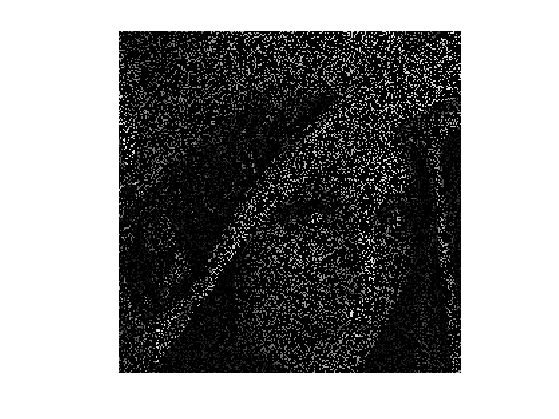## Total Variation Regularization under Constraints

We want to solve the noiseless inverse problem $$y=\Phi f$$ using a total variation regularization: $\umin{ y=\Phi f } \norm{\nabla f}_1$

This can be recasted as the minimization of $$F(K(f)) + G(f)$$ by introducing $G(f)=i_H(f), \quad F(u)=\norm{u}_1 \qandq K=\nabla,$ where $$H = \enscond{x}{\Phi(x)=y}$$ is an affine space, and $$i_H$$ is the indicator function $i_H(x) = \choice{ 0 \qifq x \in H, \\ +\infty \qifq x \notin H. }$

Shorcut for the operators.

K  = @(f)grad(f);
KS = @(u)-div(u);


Shortcut for the TV norm.

Amplitude = @(u)sqrt(sum(u.^2,3));
F = @(u)sum(sum(Amplitude(u)));


The proximal operator of the vectorial $$\ell^1$$ norm reads $\text{Prox}_{\lambda F}(u) = \max\pa{0,1-\frac{\la}{\norm{u_k}}} u_k$

ProxF = @(u,lambda)max(0,1-lambda./repmat(Amplitude(u), [1 1 2])).*u;


Display the thresholding on the vertical component of the vector.

t = -linspace(-2,2, 201);
[Y,X] = meshgrid(t,t);
U = cat(3,Y,X);
V = ProxF(U,1);
clf;
surf(V(:,:,1));
colormap jet(256);
view(150,40);
axis('tight');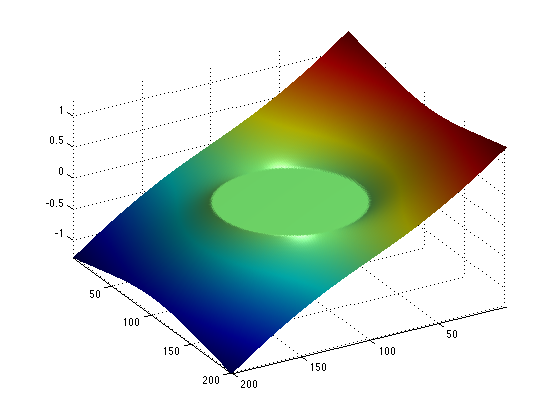For any 1-homogeneous convex functional, the dual function is the indicator of a convex set. For the $$\ell^1$$ norm, it is the indicator of the $$\ell^\infty$$ ball $F^* = i_{\norm{\cdot}_\infty \leq 1} \qwhereq \norm{u}_\infty = \umax{i} \norm{u_i}.$

The proximal operator of the dual function is hence a projector (and it does not depend on $$\sigma$$ ) $\text{Prox}_{\sigma F^*}(u) = \text{Proj}_{\norm{\cdot}_\infty \leq 1}(u).$

A simple way to compute the proximal operator of the dual function $$F^*$$, we make use of Moreau's identity: $x = \text{Prox}_{\tau F^*}(x) + \tau \text{Prox}_{F/\tau}(x/\tau)$

ProxFS = @(y,sigma)y-sigma*ProxF(y/sigma,1/sigma);


Display this dual proximal on the vertical component of the vector.

V = ProxFS(U,1);
clf;
surf(V(:,:,1));
colormap jet(256);
view(150,40);
axis('tight');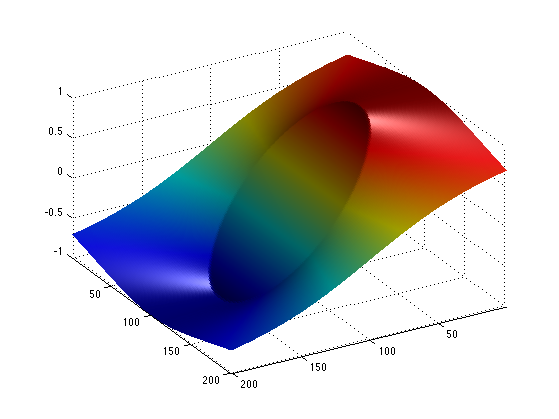The proximal operator of $$G = i_H$$ is the projector on $$H$$. In our case, since $$\Phi$$ is a diagonal so that the projection is simple to compute $\text{Prox}_{\tau G}(f) = \text{Proj}_{H}(f) = f + \Phi(y - \Phi(f))$

ProxG = @(f,tau)f + Phi(y - Phi(f));


## Primal-dual Total Variation Regularization Algorithm

Now we can apply the primal dual scheme to the TV regularization problem.

We set parameters for the algorithm. Note that in our case, $$L=\norm{K}^2=8$$. One should has $$L \sigma \tau < 1$$.

L = 8;
sigma = 10;
tau = .9/(L*sigma);
theta = 1;


Initialization, here f stands for the current iterate $$f_k$$, g for $$g_k$$ and f1 for $$\tilde f_k$$.

f = y;
g = K(y)*0;
f1 = f;


Example of one iterations.

fold = f;
g = ProxFS( g+sigma*K(f1), sigma);
f = ProxG(  f-tau*KS(g), tau);
f1 = f + theta * (f-fold);


Exercice 1: (check the solution) Implement the primal-dual algorithm. Monitor the evolution of the TV energy $$F(K(f_k))$$ during the iterations. Note that one always has $$f_k \in H$$ so that the iterates satisfies the constraints.

exo1;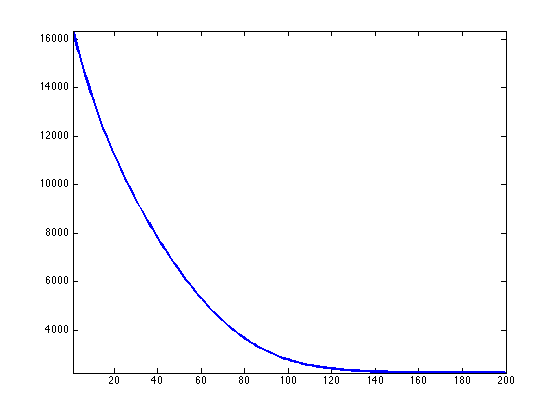Display inpainted image.

clf;
imageplot(f);Exercice 2: (check the solution) Use the primal dual scheme to perform regularization in the presence of noise $\umin{\norm{y-\Phi(f)} \leq \epsilon} \norm{\nabla f}_1.$

exo2;


## Inpainting Large Missing Regions

It is possible to consider a more challening problem of inpainting large missing regions.

To emphasis the effect of the TV functional, we use a simple geometric image.

n = 64;
name = 'square';


We remove the central part of the image.

a = 4;
Lambda = ones(n);
Lambda(end/2-a:end/2+a,:) = 0;
Phi = @(f)f.*Lambda;


Display.

clf;
imageplot(f0, 'Original', 1,2,1);
imageplot(Phi(f0), 'Damaged', 1,2,2);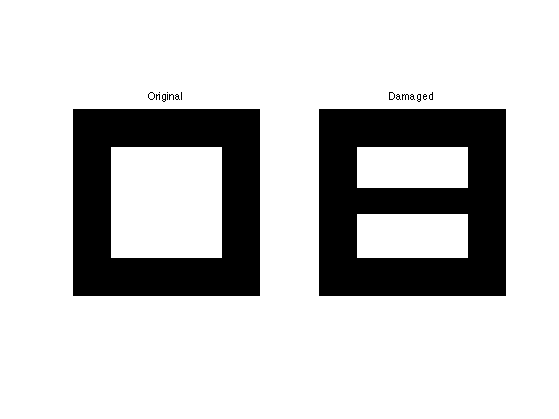Exercice 3: (check the solution) Display the evolution of the inpainting process.

exo3;